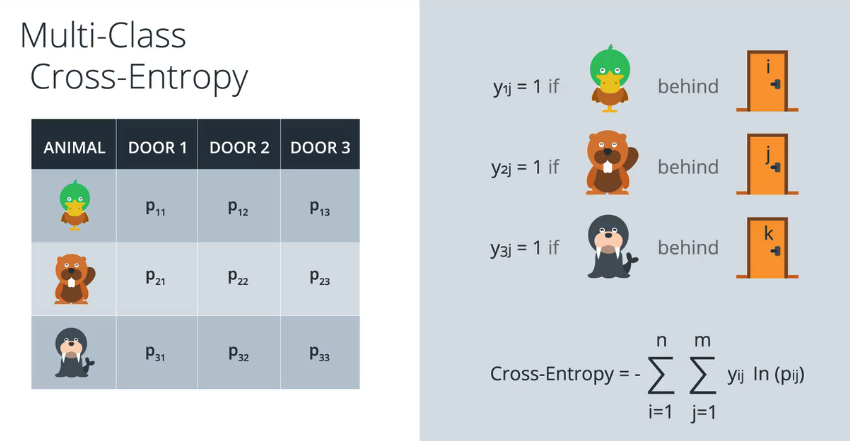# Python:交叉熵-损失函数 (六十)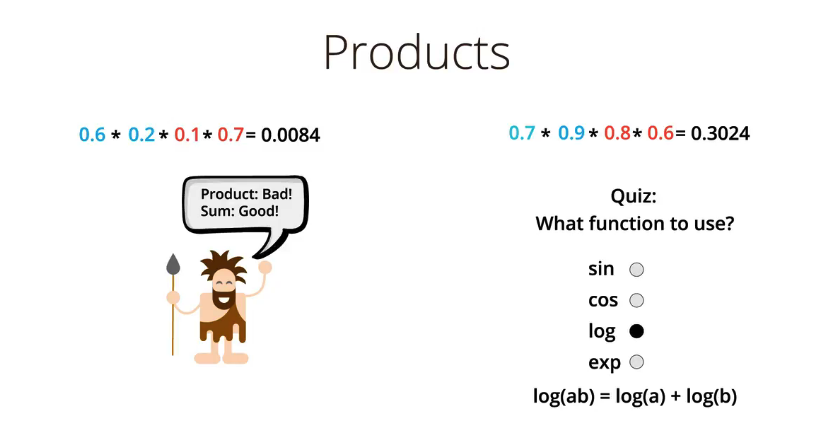e在科学技术中用得非常多，一般不使用以10为底数的对数。以e为底数，许多式子都能得到简化，用它是最“自然”的，所以叫“自然对数”。

(1+1/n)^n，n越来越大，就越接近它

e是自然对数的底数，是一个无限不循环小数，其值是2.71828……，是这样定义的：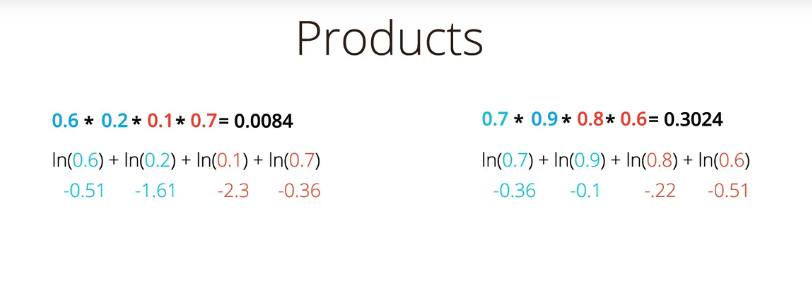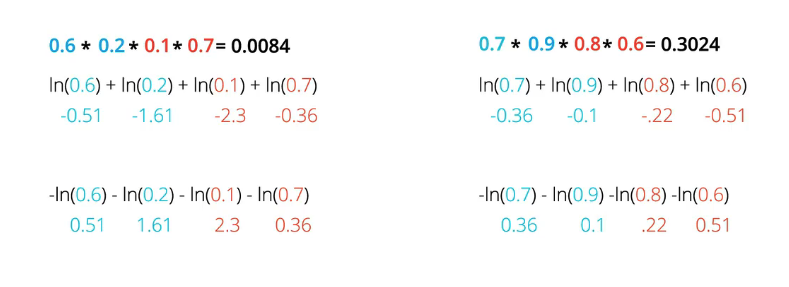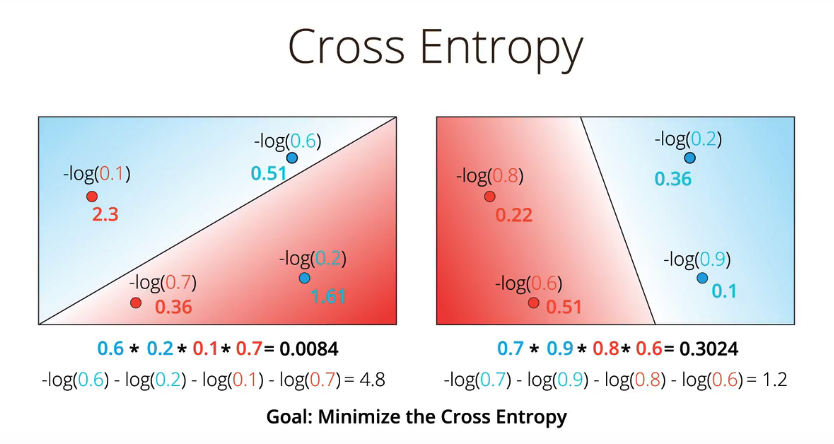## 交叉熵(cross entropy)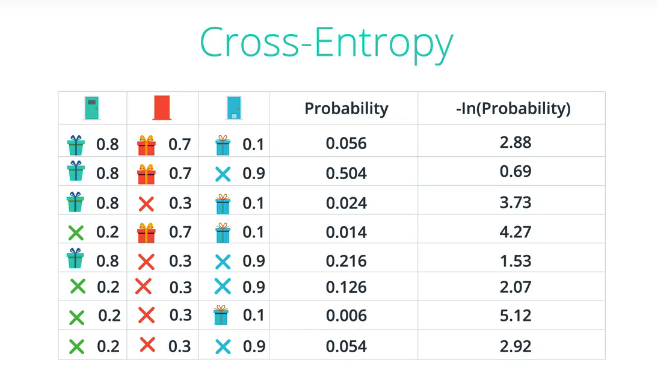### 交叉熵公式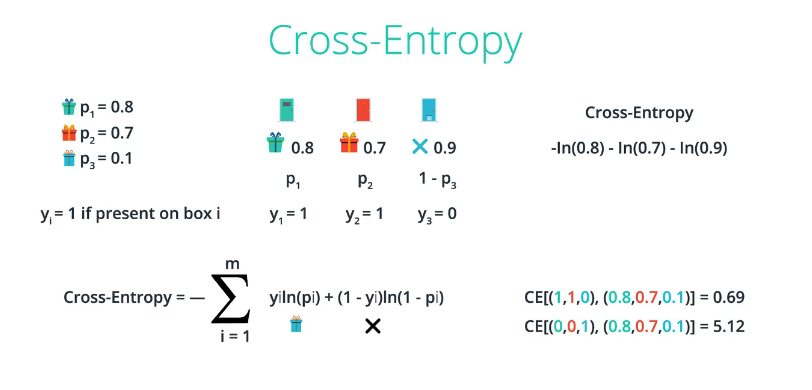CE[(1,1,0),(0.8,0.7,0.1)] = 0.69 说明：(1,1,0)表示概率分布，即是否有礼物
CE[(0,0,1),(0.8,0.7,0.1)] = 5.12

import numpy as np

# Write a function that takes as input two lists Y, P,
# and returns the float corresponding to their cross-entropy.
def cross_entropy(Y, P):
Y = np.float_(Y)
P = np.float_(P)

return -np.sum(Y * np.log(P) + (1 - Y) * np.log(1 - P))


Trying for Y=[1,0,1,1] and P=[0.4,0.6,0.1,0.5].
Correct!